Need Help?

Subscribe to Circuit

###### \${selected_topic_name}
• Notes
• Comments & Questions

$\begin{array}{l}{\text { The switch in the circuit shown in Fig, has been open for a long time. The initial charge on the }} \\ {\text { capacitor is zero. At } t=0 \text { , the switch is closed. }}\end{array}$

$\begin{array}{l}{\text { Find the expression for }} \\ {\text { a) } i(t) \text { for } t>0} \\ {\text { b) } v(t) \text { when } t>0}\end{array}$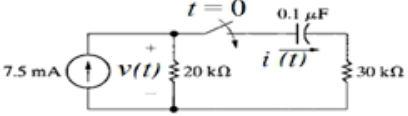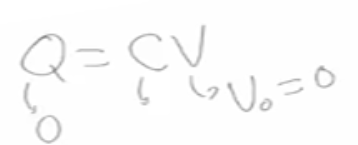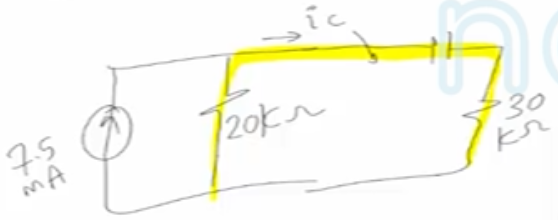$i\left(0^{+}\right)$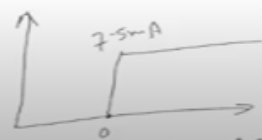$\text { Step response }$

$i_{c}\left(0^{+}\right)=\frac{7.5 *{20}}{20+30}=3 \mathrm{mA}$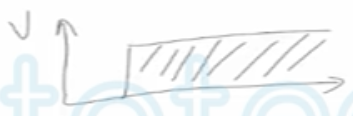$\frac{d v}{dt}=0, \quad i=c * 0=0$

$C_0 P \Rightarrow 0 \cdot c$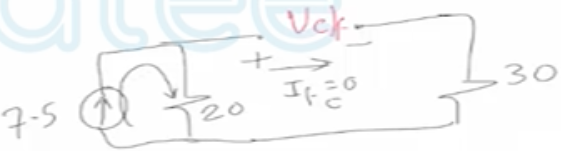$V_{c/f}=7 \cdot 5 * 10^{-3} * 20 * 10^3=150 \ volt$

$I_{c/f}=0$

$\tau=R C=50 \times 10^{3} \times0.1 \times 10^{-6}=5 \times 10^{-3} \mathrm{sec}$

$Un kown(t)=Final+[Initial-Final]e^{\frac{-(t-switch \ time)}{2}}$

$V_{c}(t)=150+[0-150] e^{-\frac{(t-0)}{5*10^{-3}}}$

$V_{c}(t)=150[1-e^{200t}] \quad \ t \geq 0$

${i_{c}(t)=0+\left[3-0\right] e^{\frac{-t}{5 \times 10^{-3}}}}$

$i_{c}(t)=3 e^{-200t} \ \quad (mA)$

No comments yet